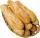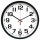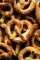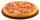# Fraction calculator

The calculator performs basic and advanced operations with fractions, expressions with fractions combined with integers, decimals, and mixed numbers. It also shows detailed step-by-step information about the fraction calculation procedure. Solve problems with two, three, or more fractions and numbers in one expression.

## Result:

### 6 + 0.413 + 0.173 = 3293/500 = 6 293/500 = 6.586

Spelled result in words is three thousand two hundred ninety-three five-hundredths (or six and two hundred ninety-three five-hundredths).

### How do you solve fractions step by step?

1. Conversion a decimal number to a fraction: 0.413 = 413/1000 = 413/1000

a) Write down the decimal 0.413 divided by 1: 0.413 = 0.413/1
b) Multiply both top and bottom by 10 for every number after the decimal point. (For example, if there are two numbers after the decimal point, then use 100, if there are three then use 1000, etc.)
0.413/1 = 4.13/10 = 41.3/100 = 413/1000
Note: 413/1000 is called a decimal fraction.

c) Simplify and reduce the fraction
413/1000 = 413 * 1/1000 * 1 = 413 * 1/1000 * 1
2. Add: 6 + 0.413 = 6/1 + 413/1000 = 6 · 1000/1 · 1000 + 413/1000 = 6000/1000 + 413/1000 = 6000 + 413/1000 = 6413/1000
For adding, subtracting, and comparing fractions, it is suitable to adjust both fractions to a common (equal, identical) denominator. The common denominator you can calculate as the least common multiple of both denominators - LCM(1, 1000) = 1000. In practice, it is enough to find the common denominator (not necessarily the lowest) by multiplying the denominators: 1 × 1000 = 1000. In the following intermediate step, it cannot further simplify the fraction result by canceling.
In other words - six plus four hundred thirteen one-thousandths = six thousand four hundred thirteen one-thousandths.
3. Conversion a decimal number to a fraction: 0.173 = 173/1000 = 173/1000

a) Write down the decimal 0.173 divided by 1: 0.173 = 0.173/1
b) Multiply both top and bottom by 10 for every number after the decimal point. (For example, if there are two numbers after the decimal point, then use 100, if there are three then use 1000, etc.)
0.173/1 = 1.73/10 = 17.3/100 = 173/1000
Note: 173/1000 is called a decimal fraction.

c) Simplify and reduce the fraction
173/1000 = 173 * 1/1000 * 1 = 173 * 1/1000 * 1
4. Add: the result of step No. 2 + 0.173 = 6413/1000 + 0.173 = 6413/1000 + 173/1000 = 6413 + 173/1000 = 6586/1000 = 2 · 3293/2 · 500 = 3293/500
For adding, subtracting, and comparing fractions, it is suitable to adjust both fractions to a common (equal, identical) denominator. The common denominator you can calculate as the least common multiple of both denominators - LCM(1000, 1000) = 1000. In practice, it is enough to find the common denominator (not necessarily the lowest) by multiplying the denominators: 1000 × 1000 = 1000000. In the following intermediate step, cancel by a common factor of 2 gives 3293/500.
In other words - six thousand four hundred thirteen one-thousandths plus one hundred seventy-three one-thousandths = three thousand two hundred ninety-three five-hundredths.

#### Rules for expressions with fractions:

Fractions - simply use a forward slash between the numerator and denominator, i.e., for five-hundredths, enter 5/100. If you are using mixed numbers, be sure to leave a single space between the whole and fraction part.
The slash separates the numerator (number above a fraction line) and denominator (number below).

Mixed numerals (mixed fractions or mixed numbers) write as integer separated by one space and fraction i.e., 1 2/3 (having the same sign). An example of a negative mixed fraction: -5 1/2.
Because slash is both signs for fraction line and division, we recommended use colon (:) as the operator of division fractions i.e., 1/2 : 3.

Decimals (decimal numbers) enter with a decimal point . and they are automatically converted to fractions - i.e. 1.45.

The colon : and slash / is the symbol of division. Can be used to divide mixed numbers 1 2/3 : 4 3/8 or can be used for write complex fractions i.e. 1/2 : 1/3.
An asterisk * or × is the symbol for multiplication.
Plus + is addition, minus sign - is subtraction and ()[] is mathematical parentheses.
The exponentiation/power symbol is ^ - for example: (7/8-4/5)^2 = (7/8-4/5)2

#### Examples:

subtracting fractions: 2/3 - 1/2
multiplying fractions: 7/8 * 3/9
dividing Fractions: 1/2 : 3/4
exponentiation of fraction: 3/5^3
fractional exponents: 16 ^ 1/2
adding fractions and mixed numbers: 8/5 + 6 2/7
dividing integer and fraction: 5 ÷ 1/2
complex fractions: 5/8 : 2 2/3
decimal to fraction: 0.625
Fraction to Decimal: 1/4
Fraction to Percent: 1/8 %
comparing fractions: 1/4 2/3
multiplying a fraction by a whole number: 6 * 3/4
square root of a fraction: sqrt(1/16)
reducing or simplifying the fraction (simplification) - dividing the numerator and denominator of a fraction by the same non-zero number - equivalent fraction: 4/22
expression with brackets: 1/3 * (1/2 - 3 3/8)
compound fraction: 3/4 of 5/7
fractions multiple: 2/3 of 3/5
divide to find the quotient: 3/5 ÷ 2/3

The calculator follows well-known rules for order of operations. The most common mnemonics for remembering this order of operations are:
PEMDAS - Parentheses, Exponents, Multiplication, Division, Addition, Subtraction.
BEDMAS - Brackets, Exponents, Division, Multiplication, Addition, Subtraction
BODMAS - Brackets, Of or Order, Division, Multiplication, Addition, Subtraction.
GEMDAS - Grouping Symbols - brackets (){}, Exponents, Multiplication, Division, Addition, Subtraction.
Be careful, always do multiplication and division before addition and subtraction. Some operators (+ and -) and (* and /) has the same priority and then must evaluate from left to right.

## Fractions in word problems:

• Fraction and a decimalWrite as a fraction and a decimal. One and two plus three and five hundredths
• One third 2One third of all students in class live in a house. If here are 42 students in a class, how many of them live in house?
• RollsMom bought 13 rolls. Dad ate 3.5 rolls. How many rolls were left when Peter yet ate two at dinner?
• AkpanAkpan spent 3/8 of his time in school during the week. What fraction of his time does he spend at home during the week?
• Missing numberBlank +1/6 =3/2 find the missing number
• Two thirdsTwo thirds of the unknown number is 8/7. What is 7/3 of this number?
• Mixed numbersRewrite mixed numbers, so the fractions have the same denominator: 5 1/5 - 2 2/3
• A class IV.CIn a class 2/5 were boys. 30 were girls. How many more girls than boys are there?
• The book 4Mr. Kinion read 3 3/4 chapters in his book on Monday. He then read 2 4/6 more chapters on Tuesday. How many chapters has he read so far?
• Max hasMax has a 1/3 of a pound of pretzels and wants to share equal parts between himself and a friend. How much pretzels will Max's friend receive?
• One largeOne large pizza is cut into 12 pieces. The pizza has sausage, mushrooms, and cheese on it. Michelle ate 1/6 of the pizza, Natalie ate 1/4 of the pizza, Bridgette ate 1/8 of the pizza, and Brenda ate 1/3 of the pizza. How much of the whole pizza is left, a
• A pizza 2A pizza is cut into slices that are each 1/6 of the whole. John is going to eat 1/2 of the whole pizza. How many slices will John eat?
• Land areaThe land area of Asia and Africa are in a 3: 2 ratio, the European and African are is 1:3. What are the proportions of Asia, Africa, and Europe?